# How to Add Prefix or suffix to Cell

This post will guide you how to add common prefix or suffix to all cells or the range of cells in your worksheet in Excel. How do I add the same prefix or suffix to a range of cells without any changing the existing numbers or text in the range. How to add prefix or suffix to all cells with Excel VBA Macro.

Assuming that you have a list of data that you want to add common text to the starting position of all cells, of course, you can do it by manually, but it will consume lots of time and it is not a good idea. And you can use the concatenate operator, the CONCATENATE function to create a new Excel formula to add prefix or suffix to the range of cells that you selected. Or you can write a new Excel VBA Macro to achieve the same result.

## Method 1: Using Concatenation Operator to add prefix or suffix

If you want to add prefix or same text to the starting of all cells in your range, you can use the Concatenation operator to build a formula, for example, you want to add same text “excelhow” to all cells in range A1:A4, then you can use the following formula:

`="excelhow"&A1`

Ether this formula in a blank cell, such as: B1, then drag the AutoFill Handle down to other cells to apply this formula.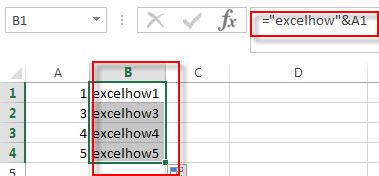If you want to add suffix to all cells with concatenation operator, you can use the following formula:

`=A1&"excelhow"`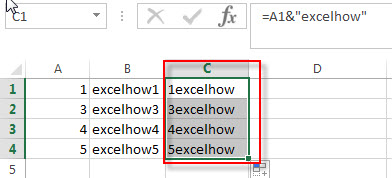You will see that all cells are added the same prefix text in your worksheet.

## Method 2: Using concatenation function to add prefix

To add prefix or suffix text or number to the range of cells with concatenation function, you can wirte down the following formula:

```=CONCATENATE("excelhow",A1)
or
=CONCATENATE(A1,"excelhow")```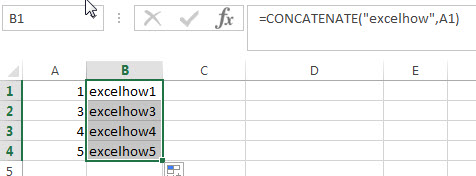## Method 3: Using Excel VBA Macro to add prefix

You can also use the following VBA Macro code to add prefix or suffix in your worksheet in Excel.  Let’s see the below steps:

1# click on “Visual Basic” command under DEVELOPER Tab.2# then the “Visual Basic Editor” window will appear.

3# click “Insert” ->”Module” to create a new module4# paste the below VBA code into the code window. Then clicking “Save” button.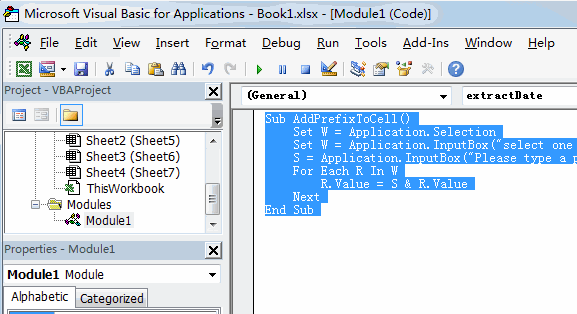```Sub AddPrefixToCell()
Set W = Application.Selection
Set W = Application.InputBox("select one Range that you want to add prefix to each cell:", "AddPrefixToCell", W.Address, Type:=8)
For Each R In W
R.Value = S & R.Value
Next
End Sub```

5# back to the current worksheet, then run the above excel macro. Click Run button.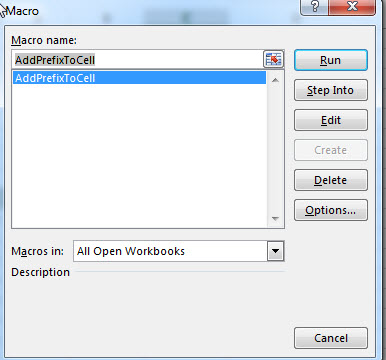6# select a range that you want to add prefix, click OK button, then add one prefix string or character. Click OK button.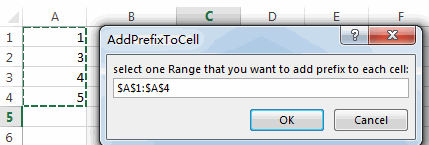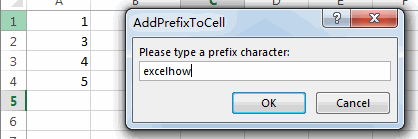7# you will see that the prefix is added to all range of cells.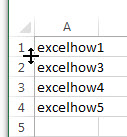If you want to add suffix to a range of cells with VBA macro in Excel, you can use the following VBA macro.

```Sub AddSuffixToCell()
Set W = Application.Selection
Set W = Application.InputBox("select one Range that you want to add suffix to each cell:", "AddSuffixToCell", W.Address, Type:=8)
For Each R In W
R.Value = R.Value & S
Next
End Sub```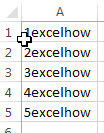### Related Functions

• Excel Concat function
The excel CONCAT function combines 2 or more strings or ranges together.This is a new function in Excel 2016 and it replaces the CONCATENATE function.The syntax of the CONCAT function is as below:=CONCAT (text1,[text2],…)…

Related Posts

Find and Replace Multiple Values

This post will guide you how to find and replace multiple values at once with VBA macro or using formula  in Excel. How do I make multiple find and replace in Excel. Suppose that you have a few cells containing ...

VBA Macro For VLOOKUP From Another Sheet

In the previous post, you should know that how to fix or remove the #N/A error when using VLOOKUP formula to lookup value from another sheet. And this post will show you how to use VBA code to vlookup data ...

How To Insert Comments in Protected Worksheet in Excel

This post will show you how to allow comments in a protected worksheet in Excel. You can easily to insert comments into cells in a normal worksheet in Excel, but if want to insert a comment in a worksheet that ...

How To Convert Text to Upper Cases(Using VBA) in Excel

This post will show you how to switch from lower case to upper case in Excel. and I am going to show you two different ways of converting text to upper cases using formula or VBA macro in Excel 2013,Excel ...

How To Hide Every Other Row in Excel (Using VBA)

This post will show you how to hide alternate rows or columns in Excel or how to hide every third, fourth, fifth row or column in Excel. If you want to hide every other row in your current worksheet, how ...

How to Disable the Save As Prompt in Excel

This post will show you how to use a VBA Macro to save an Excel file and overwrite any existing file without a prompt so that you are going to get the little window that says file already exists do ...

How to Count Cells that Contain even or odd numbers in Excel

This post will guide you how to count the number of cells that contain odd or even numbers within a range of cells using a formula in Excel 2013/2016.How do I count cells that contain odd numbers through the use ...

How to Count Cells that Contain negative Numbers in Excel

This post will guide you how to count the number of cells that contain negative numbers within a range of cells using a formula in Excel 2013/2016.You can count the number of negative numbers in your data using easy functions ...

How to Count Cells Are Not Blank or Empty in Excel

This post will guide you how to count cells that are not blank or empty in a given range cells using a formula in Excel 2013/2016.How do I count the number of cells that are not blank in a particular ...

How to Count Cells Not equal to X or Y in Excel

This post will guide you how to count the number of cells not equal to criteria X or Y in a given range cells using a formula in Excel 2013/2016.You can easily to count cells equal to or not equal ...

Sidebar